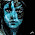### Rational Decisions

Week 6 of Introduction to Mathematical Philosophy is about rational decision making.

There are a number of possible acts an agent can choose, and associated with each act are a number of possible outcomes, each with a certain probability. Each outcome has an associated utility value which specifies how much the agent desires the corresponding outcome. Given these parameters, it is possible to calculate which act results in the maximum utility, and the Principle of Maximizing Expected Utility asserts that a rational agent will act in the manner which maximizes the expected utility.

These are 4 axioms of rational decision making. These axioms lead to the theorem that every decision of a VNM-rational agent in a given set of scenarios is characterized by maximization of the expected value of a single function u, which is the utility function.

Not surprisingly, real life human decisions often violate the VNM theorem, such that no single utility function can explain every preference in a given set of scenarios. Example of this include the Allais paradox and the Ellsberg paradox.